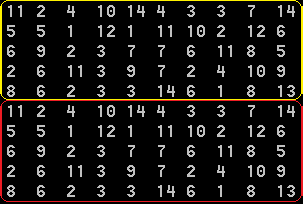﻿ Use of rand() in OpenMP parallel sections# Use of rand() in OpenMP parallel sections

I came across an interesting thread at RSDN forum where a specific error of rand() function use in OpenMP parallel sections is considered (http://rsdn.org/forum/cpp.applied/3400925.flat). I collect various errors which deal with OpenMP technology use so that to implement their troubleshooting in VivaMP static code analyzer in future. The error considered at the forum is perhaps a very specific one to implement a rule for its verification, so I decided just to write about it in the blog.

The error consists in the fact that every parallel thread has its own seed and if no special initialization is carried out, rand() function will return the same value in all the threads. Most likely, this will not be the required result.

Note: seed is initial value given to the random sequence generator in order to obtain the first random number. If you assign seed a particular value, the numbers sequence will always repeat starting with this very number.

The following code example is given at the forum:

``````void initMatrix(int** m, int H, int W)
{
#pragma omp parallel
{
#pragma omp for
for (int i = 0; i < H; ++i)
for (int j = 0; j < W; ++j)
m[i][j] = rand()%15;
}
}``````

The result of such code work is filling of matrix with repeating blocks of numbers. For example, a 10×10 matrix filled in in two threads can look as follows:As we can see, the upper and the lower parts of the matrix filled in in two different threads are the same.

In order to avoid the same rand() function behavior, in your code, you should initialize random numbers generator in each parallel thread with various values. To do this, the combination of current time from the current thread number can be used.

The corrected code will look as follows:

``````void initMatrix(int** m, int H, int W)
{
#pragma omp parallel
{
#pragma omp for
for (int i = 0; i < H; ++i)
for (int j = 0; j < W; ++j)
m[i][j] = rand()%15;
}
}``````##### Use PVS-Studio to search for bugs in C, C++, C# and Java

We offer you to check your project code with PVS-Studio. Just one bug found in the project will show you the benefits of the static code analysis methodology better than a dozen of the articles.

409
14 072

### Do you make errors in the code?